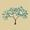Introduction to Computation Theory: Heuristics

Title:
Introduction to Computation Theory: Heuristics
Description:

These videos are from the Introduction to Computation course on Complexity Explorer (complexityexplorer.org) taught by Prof. Josh Grochow. This course is an overview of some basic principles of computation and computational complexity, with an eye towards things that might actually be useful without becoming a researcher.

Students who take this course should have basic knowledge of the principles of graphs. Some tutorial material references linear algebra, but familiarity is not necessary. This tutorial uses proofs, and requires understandings of formal math notations.

more » « less
Video Language:
English
Team:Complexity Explorer
Project:
Introduction to Computation Theory
Duration:
07:59ComplexityExplorer moved Introduction to Computation Theory: Heuristics to Complexity ExplorerComplexityExplorer added a video: Introduction to Computation Theory: Heuristics